# Scalar curvature

Jump to: navigation, search

of a Riemannian manifold at a pointThe trace of the Ricci tensor with respect to the metric tensor. The scalar curvature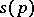is connected with the Ricci curvatureand the sectional curvatureby the formulas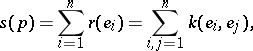where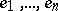is an orthonormal basis of the tangent space. In the equivalent Einstein notation, these equations have the form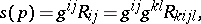where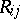and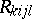are the components of the Ricci tensor and the curvature tensor, respectively, and the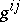are the contravariant components of the metric tensor.